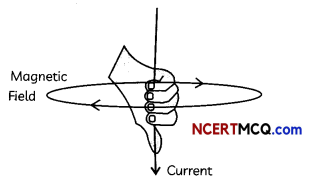## Magnetic Field Due to a Current Carrying Straight Conductor

A current carrying conductor has a magnetic field associated with it, which was first demonstrated by H. C. Oersted. Let us understand what determines the pattern of the magnetic field generated by a current through a conductor.

Example 1.
Case Based:
Take a battery (12 V), a variable resistance (or a rheostat), an ammeter (0-5 A), a plug key, and a long straight thick copper wire. Insert the thick wire through the center, normal to the Stane of rectangular cardboard. Take care that the cardboard is fixed and does not slide up or down. Connect the copper wire vertically between points X and Y in series with the battery, a plug and key.

Sprinkle some iron filings uniformly on the cardboard.

Keep the variable of the rheostat at a fixed position and note the current through the ammeter.

Close the key so that a current flows through the wire. Ensure that the copper wire placed between the points X and Y remain vertically straight Gently tap the cardboard a few times. Observe the pattern of the iron filings.
Place a compass at a point (say P) over a circle and observe the direction of the needle. Show the direction by an arrow.
(A) Select the correct observations when the card-board in above activity is gently tapped after closing the key:
(I) The iron filings align themselves in a pattern of concentric circles around the copper wire.
(II) The iron filings align themselves in straight lines around the copper wire.
(III) The concentric circles represent the mag-netic field lines.
(IV) The straight lines represent the magnetic field lines.
(a) Both (I) and (III)
(b) Both (I) and (IV)
(c) Both (II) and (III)
(d) Both (II) and (IV)
(a) Both (I) and (III)

Explanation: When the cardboard is gently tapped after closing the key, it is observed that the iron filings arrange themselves in the form of concentric circles around the straight, copper wire. These circles represent the magnetic field lines.(B) When a compass needle is placed at a point over the circle:
(a) There is no deflection observed.
(b) The direction of the south pole of the compass needle gives the direction of the field lines.
(c) The direction of the south pole of the compass needle gives the direction of the current.
(d) The direction of the north pole of the compass needle gives the direction of the field lines.
(d) The direction of the north pole of the compass needle gives the direction of the field lines.

Explanation: When a compass needle is placed at a point P over the circle, the direction of the north pole of the compass needle would give the direction of the field lines produced by the electric current through the straight wire at point P.

(C) What change is observed in the deflection of a compass needle placed at a point over the circle when direction of current flowing is reversed?
When the direction of current flowing in the wire is reversed, the deflection of the compass needle also gets reversed. This is because of the change in the direction of magnetic field produced around the current carrying wire on changing the direction of current.

Direction of magnetic field is given by Right hand thumb rule.(D) What happens to the deflection of the needle if the compass is moved from the copper wire but the current through the wire remains the same?
The deflection in the needle decreases as the magnetic field produced by a given current in the conductor decreases as the distance from it increases.

(E) Assertion (A): Deflection in the compass needle placed at a given point increases on increasing the current through the wire.
Reason (R): Copper is a good conductor of electricitg.
(a) Both (A) and (R) are true and (R) is the correct explanation of the assertion.
(b) Both (A) and (R) are true, but (R) is not the correct explanation of the assertion.
(c) (A) is true, but (R) is false.
(d) (A) is false, but (R) is true.
(b) Both (A) and (R) are true, but (R) is not the correct explanation of the assertion. Explanation: The deflection in the compass needle increases on increasing the current through the wire because the magnitude of the magnetic field produced at a given point increases as the current through the wire increases.

The field produced by the current passing through the wire depends upon the following factors:

1. It is directly proportional to the current passing in the wire.
2. It is inversely proportional to the distance from the wire.## Right-Hand Thumb Rule

Imagine that you are holding a current carrying wire in your right hand such that the thumb is stretched along the direction of the current, then, the direction in which the fingers wrap around the conductor will give the direction of the field lines of the magnetic field.Example 2.
Which of the following correctly describes the magnetic field near a long straight wire?
(a) The field consists of straight lines perpendicular to the wire.
(b) The field consists of straight lines parallel to the wire.
(c) The field consists of radial lines originating from the wire.
(d) The field consists of concentric circles centred on the wire.